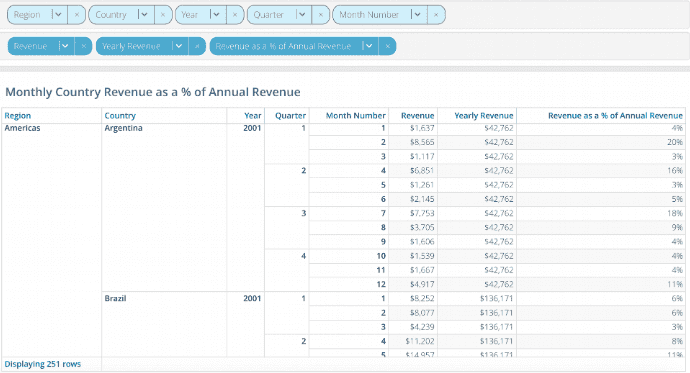You are viewing content for 5.1 | 5.0 | 4.9 | 4.8 | 4.7 | 4.6 | 4.5 | 4.4 | 4.3 | Previous Releases

# Aggregation Functions

Aggregation functions are used to summarize the data in a column. Applying them returns a single value. For example, `min` returns the smallest value in a column.

## Functions Operating on Numeric Scalars in a Column

The functions in this table operate on numeric scalars. The only allowed type is `double`.

Function Syntax Description
average() `average(double expr)` Compute the average in a column
median() `median(double expr)` Find the median in a column
min() `min(double expr)` Find the minimum value in a column
max() `max(double expr)` Find the maximum value in a column
sum() `sum(double expr)` Find the sum of the values in a column

## Functions Counting Items of Any Type in a Column

The functions in this table operate on any Incorta data type. For this table, ”<item>” stands for any Incorta data type.

Function Syntax Description
count() `count(<item>)` Returns the number of elements in a column
distinct() `distinct(<item>)` Returns the number of distinct groups of elements in a column

## Aggregation with Level Based Measure

Aggregation functions can take, as a second argument, the `groupBy` parameter. This is an optional parameter and is valuable when creating a Level based measure.

In the example below, the user wants to create an Insight to show the percentage contribution of monthly revenue to annual revenue by country and region.To do this, the user needs to create two derived formula columns:

1. Yearly Revenue, a measure aggregated at a higher level than the monthly grain and repeated for each of the monthly grain data values.
2. Revenue at the monthly grain/Yearly Revenue Alan: not sure if I got bullet number 2 correctNote the sequence of the `groupBy` dimensions in the formula is important and should match the sequence of grouping dimensions in the insight. In the insight above you will see Region, Country and then Year in the grouping dimension tray.